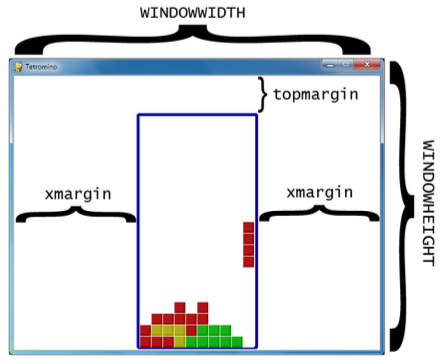# 8.6: More Setup Code

•• Al Sweigart
$$\newcommand{\vecs}{\overset { \rightharpoonup} {\mathbf{#1}} }$$ $$\newcommand{\vecd}{\overset{-\!-\!\rightharpoonup}{\vphantom{a}\smash {#1}}}$$$$\newcommand{\id}{\mathrm{id}}$$ $$\newcommand{\Span}{\mathrm{span}}$$ $$\newcommand{\kernel}{\mathrm{null}\,}$$ $$\newcommand{\range}{\mathrm{range}\,}$$ $$\newcommand{\RealPart}{\mathrm{Re}}$$ $$\newcommand{\ImaginaryPart}{\mathrm{Im}}$$ $$\newcommand{\Argument}{\mathrm{Arg}}$$ $$\newcommand{\norm}{\| #1 \|}$$ $$\newcommand{\inner}{\langle #1, #2 \rangle}$$ $$\newcommand{\Span}{\mathrm{span}}$$ $$\newcommand{\id}{\mathrm{id}}$$ $$\newcommand{\Span}{\mathrm{span}}$$ $$\newcommand{\kernel}{\mathrm{null}\,}$$ $$\newcommand{\range}{\mathrm{range}\,}$$ $$\newcommand{\RealPart}{\mathrm{Re}}$$ $$\newcommand{\ImaginaryPart}{\mathrm{Im}}$$ $$\newcommand{\Argument}{\mathrm{Arg}}$$ $$\newcommand{\norm}{\| #1 \|}$$ $$\newcommand{\inner}{\langle #1, #2 \rangle}$$ $$\newcommand{\Span}{\mathrm{span}}$$$$\newcommand{\AA}{\unicode[.8,0]{x212B}}$$

XMARGIN = int((WINDOWWIDTH - BOARDWIDTH * BOXSIZE) / 2)
TOPMARGIN = WINDOWHEIGHT - (BOARDHEIGHT * BOXSIZE) - 5


The program needs to calculate how many pixels are to the left and right side of the board to use later in the program. WINDOWWIDTH is the total number of pixels wide the entire window is. The board is BOARDWIDTH boxes wide and each box is BOXSIZE pixels wide. If we subtract BOXSIZE pixels from this for each of the boxes wide in the board (which is BOARDWIDTH * BOXSIZE), we’ll have the size of the margin to the left and right of the board. If we divide this by 2, then we will have the size of just one margin. Since the margins are the same size, we can use XMARGIN for either the left-side or right-side margin.

We can calculate the size of the space between the top of the board and the top of the window in a similar manner. The board will be drawn 5 pixels above the bottom of the window, so 5 is subtracted from topmargin to account for this.#               R    G    B
WHITE       = (255, 255, 255)
GRAY        = (185, 185, 185)
BLACK       = (  0,   0,   0)
RED         = (155,   0,   0)
LIGHTRED    = (175,  20,  20)
GREEN       = (  0, 155,   0)
LIGHTGREEN  = ( 20, 175,  20)
BLUE        = (  0,   0, 155)
LIGHTBLUE   = ( 20,  20, 175)
YELLOW      = (155, 155,   0)
LIGHTYELLOW = (175, 175,  20)

BORDERCOLOR = BLUE
BGCOLOR = BLACK
TEXTCOLOR = WHITE

Each of these four colors will be stored in tuples named COLORS (for the normal colors) and LIGHTCOLORS (for the lighter colors).